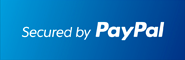** New: ECONOMICS , DIFFERENTIAL EQUATIONS **         ** SPECIALS for bulk apps **         ** Bestsellers: CALCULUS , SAT , PHYSICS , CHEM , STOCKS & PORTFOLIOS , ALGEBRA **# #1 Linear Algebra Solver for the TI-89 Calculator

## Solve Linear Algebra problems Step by Step.

### Works With TI92 Plus TI89 TI89 Titanium Voyage 200At the end of the PayPal checkout, you will be sent an email containing your key and software.Description

• Enter (Random) Matrices A and B easily (MME has its ownMatrix Editor) under F1:,
• Ideal for Matrix and Vector Classes.
• View A,B,AT, BT , even as Binary or Hexadecimalnumbers, etc
• Perform 30+ Matrix Computations (under F2) such as A+B, A-B, k*A, A*B, B*A, A-1, det(A), Eigenvalues, LU and QR - Factorization, Norm, Trace,more .
• Step by Step - Simplex Algorithm.
• Step by Step - Gaussian Elimination.
• Step by Step - Find Inverse
• Step by Step - Find Determinant
• Step by Step - Row Echelon and Reversal (REF and RREF)
• Step by Step - Gauss and Gauss Jordan Elimination
• Step by Step - Cramer Rule
• Step by Step - EigenValues and EigenVectors.
• Step by Step - Square Root Matrix
• Solve any n by n system of equations.
• Rotation Matrices, Magic Squares and much more.
• OrthoNormal Basis
• Nullity, Null-, Row- and ColumnSpace Basis.
• Cross and Dot Product, UnitVector, Angle between Vectors
• Projection A onto B, Distance A to B, etc ...
• Vector Differentiation and Integration
• Test Orthogonality, Find Orthogonal Vector, Test Independence

Visit the Classic Site at www.ti89.com/index.htm for more details.

Documentation